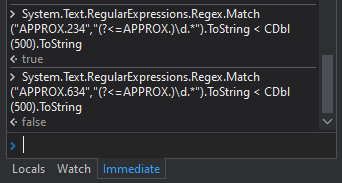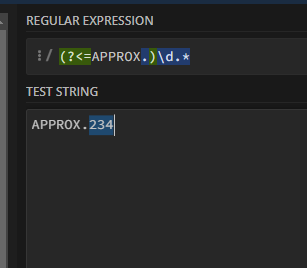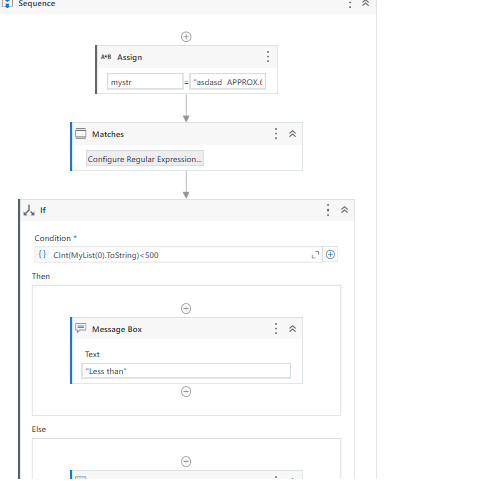# Extract and compare text

The value of get text is a paragraph where there will be a 3 digit number next to the word “APPROX.” . I need to check if the 3 digit number is less than 500. How do i write this ? Also this is in a loop hence i have to give the 3 digit number a variable.

You can use Regex expression?

You can try this expression in IF activity

``````System.Text.RegularExpressions.Regex.Match("APPROX.634","(?<=APPROX.)\d.*").ToString < CDbl(500).ToString
``````Regards
Gokul

I need to make the 3 digit number a variable before comparing. How do i do that?

Hello @RACHEL_PAUL
You can use Matches activity and provide the expression as (\d) as in the below image.

CInt(MyList(0).ToString)<500

Above expression to convert String to Integer. MyList is the output of matches activity.Use Assign activity

``````YourVariable = System.Text.RegularExpressions.Regex.Match("APPROX.634","(?<=APPROX.)\d.*").ToString

Output -> 634
``````

Regards
Gokul

Since it is in a loop the 3 digit number doesnt stay the same so i cannot assign any value rather a variable. How do i extract this data from get text value?

Not clear for me @RACHEL_PAUL , Can you tell me what you what to achieve and share some sample input from the get text and what you want to get the string value?

In a for each loop. A paragraph is stored in a gext text variable. The paragraph contains a word “approx. ××××” where ×××× generates a new number every iteration. I need to get that value in a message box. How do i do that?